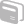# Activities

•• ##### Subject Area

• Maths: Number: Number
• Maths: Algebra: Algebra
• Maths: Multiple Representations: Multiple Representations

• ##### Author11-14
14-16

2 Hours

• TI-Nspire™

TI-Nspire™

## Trial and Improvement

#### Activity Overview

This activity allows learners to investigate the solution to an equation by trial and improvement. Learners are led through multiple representations of the approximate solution of a quadratic equation using the spreadsheet and graphing applications. The form of the quadratic is introduced using the data and statistics graphing facility.

#### Before the Activity

Some knowledge of substituting numbers in formulas and different forms of quadratic equation is required.

#### During the Activity

Objective: to investigate the solutions to a quadratic equation numerically and graphically.

#### After the Activity

This could lead into further work on the algebraic solution of quadratic equations.